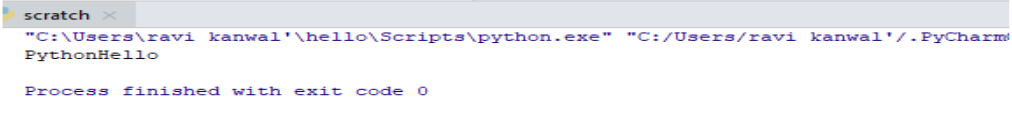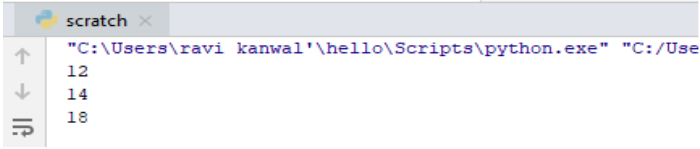×

Html & Html5

Introduction to Html

Component of Html

Html Form

Html5 basics

Html5 Grid

Html5 Graphics

Html5 Media

Html5 APIs

Python and Pandas

Introduction to Python

Component of Python

Python Function

A function is group related statement that performs the specific task.Python provide built-in function like print() etc. you create own function that is called user defined function. We can pass data ,known as parameters into a function.
Function defined in the Python using def keyword.

Syntax of Python :

def function_name(parameters):

statement()

Calling a function()

To call a function we use the function name and followed by parenthesis()

let's have an example :-

def my_function():

print("hello Python")

my_function()

Once we have create a function and we can call it from another function . To call a function we simply type the function name with appropriate parameters.

Parameters

Information can be passed to a function known as a parameters.parameters specified after the function name,and inside the parentheses.you can add the more parameter as we want,just separate them with comma.

let's have an example :-

def my_function(fname):

print(fname+ "Hello" )

my_function("Python")

Output of this ProgramSo just by calling the function we get our desired output

Default Parameter Values

If we are call function without any parameter thats is uses the default values .

let's have an example :-

def myFun(x, y=15):

print("x: ", x)

print("y: ", y)

myFun(20)

Output of this Programhere we just assign the value to 'x' but in the output we get the default value of 'y' too.

Passing a List as a parameters

We can send the all data type parameter to a function such as a (String,Number,list,Dictionary etc..) it will be created same data type in a function.

let's have an example :-

def my_function(food):

for x in food:

print(x)

fruits = ["Banana", "Mango", "Apple"]

my_function(fruits)

Output of this Programhere we just get the list in sequence just bvy the calling the function.

Return value

The return statement with no argument is the same as return none.To let function return we can use return statement.

let's have an example :-

def my_fun(y):

return 9 + y

print(my_fun(3))

print(my_fun(5))

print(my_fun(9))

Output of this ProgramSo here in return we are getting 9 added to the values we assigned at the time of calling function.

Recursion

Recursion is process to defining something in terms of itself.

let's have an example :-

def calc_factorial(x):

if x == 1:

return x

else:

return (x * calc_factorial(x-1))

num =5

print("The factorial of", num, "is", calc_factorial(num))

Output of this ProgramSo here we get the factorial value of 5.

python training insitute| Best IT Training classes in Gurgaon | python training